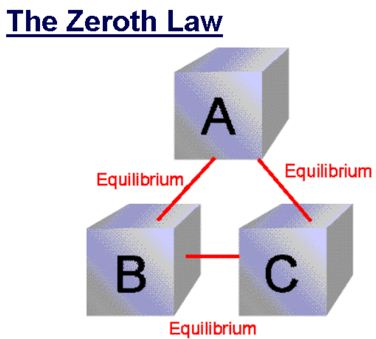Physics

# Explain Zeroth Law of Thermodynamics

If two systems A and B are separately in thermal equilibrium with a third system C, then the three systems are in thermal equilibrium with each other Zeroth law of thermodynamics states that two systems which are individually in thermal equilibrium with a third one, are also in thermal equilibrium with each other.The Zeroth Law of Thermodynamics states that if two bodies are each in thermal equilibrium with some third body, then they are also in equilibrium with each other. This Zeroth law was stated by Flower much later than both first and second laws of thermodynamics. This law helps us to define temperature in a more rigorous manner.